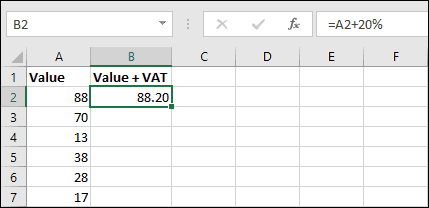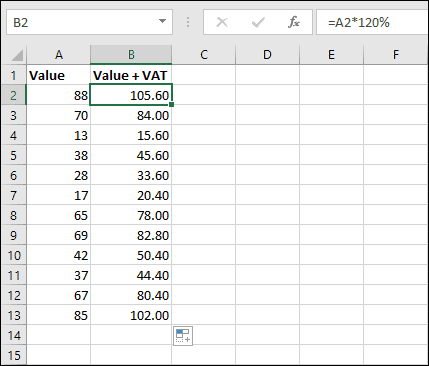# Adding VAT to a value in Excel

Sales taxes are levied in many parts of the world. In the UK, this is known as VAT, or “Value-added Tax”, but it’s also known as sales tax, consumption tax and GST (Goods and Services Tax).

Adding VAT to values is a very common requirement, but doing this in Excel isn’t very intuitive and confuses many users.

Your first instinct to add 20% VAT to a number might be to use a formula like this:

=A2+20%Unfortunately, this doesn’t work. If you’ve read our earlier article about subtracting percentages, you already know why.

If you’re completely new to Excel and have no understanding of formulas, you can learn how to create simple formulas in our completely free Basic Skills course. I’d recommend starting there if formulas are completely new to you. Otherwise, read on for the solution.

## Percentages are actually decimal values

When you type 20% into Excel, Excel sees it as the value 0.2. Excel does this with all percentage values. 50% is 0.5, 75% is 0.75, and so on.

In the previous example, you were actually asking excel to add 0.2 to the value instead of adding 20%, resulting in 88.20 instead of the correct result, which would be 105.6.

## The solution

So, knowing how Excel treats percentages, how should I add the 20% VAT?

To get your value plus 20%, you actually want to get 120% of the original value, so one solution is to multiply the number by 120% with one of the following formulas:

=A2*120%
=A2*1.2

Because of the way Excel handles percentages, it sees 120% and 1.2 as exactly the same value.

An alternative, but more long-winded calculation, would be to calculate 20% of the number and then add it to the original number, with one of these formulas:

=A2+(A2*20%)
=A2+(A2*0.2)

This will have exactly the same result and, as in the previous example, both of these formulas mean exactly the same thing to Excel.Once you’ve written the formula you can easily fill it down to as many records as you need. AutoFilling formulas is explained in depth in our free Basic Skills course.

Our Essential Skills course continues from Basic Skills and explains Excel’s handling of percentages in greater depth, as well as many other features. I’d highly recommend it if you want to improve your Excel skills even further.

### 4 thoughts on “Adding VAT to a value in Excel”

1.thanks for this! I got to do it right!!!

2.Wow, except this formula doesn’t actually work, on account of the fact that you are adding 20% to the net value, when vat is 20% of the gross value. The two are not the same.

If VAT is charged at 20%. That means that if you have a gross value of 105.6, as in the example, the VAT would be 21.12 (20% of 105.6) and the net amount would be 84.48 (80% of 105.6).

1.Hi Mark,

I think you might be misunderstanding what this article is trying to show – the example is not trying to calculate the VAT amount from a net value, it is simply adding the VAT to a value that has not yet had the tax applied.

It sounds like you’re talking about trying to calculate how much VAT has been added to a value that has already had VAT applied. That is not what this article covers, but that kind of calculation (using a different example) is covered in this article.

3.or moreover…he is going to have a problem if he is in business since it is 20% of the net!

Scroll to Top